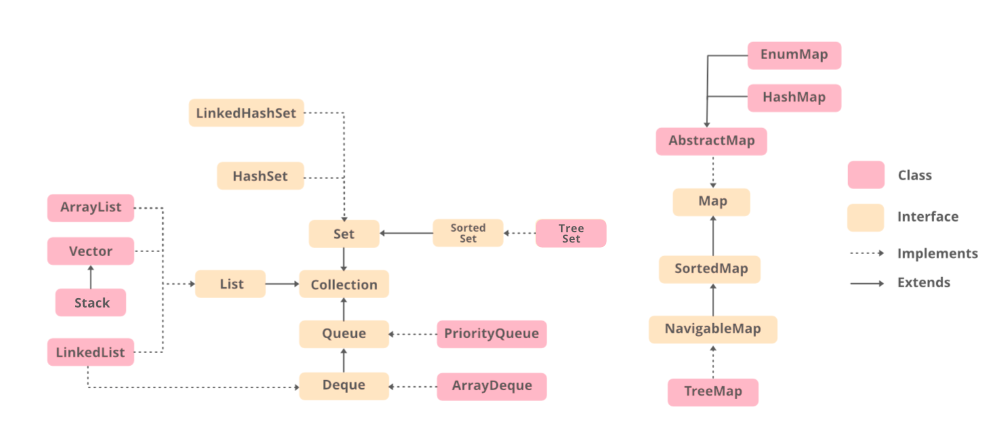# Difference between Arrays and Collection in Java

• Difficulty Level : Basic
• Last Updated : 29 Dec, 2021

An array in Java is a group of like-typed variables referred to by a common name. Arrays in Java work differently than they do in C/C++. Following are some important points about Java arrays. On the other hand, any group of individual objects which are represented as a single unit is known as the collection of the objects. In Java, a separate framework named the “Collection Framework” has been defined in JDK 1.2 which holds all the collection classes and interface in it.

The most essential thing while dealing Collection is to have super strong grasp of Collection framework which in one go is depicted via below image as follows:Example

## Java

 `// Java Program to Illustrate Difference``// Between Arrays and Collection` `// Importing required classes``import` `java.util.*;` `// Main class``class` `GFG {` `    ``// Main driver method``    ``public` `static` `void` `main(String[] args)``    ``{` `        ``// Arrays``        ``String[] gfg``            ``= ``new` `String[] { ``"G"``, ``"E"``, ``"E"``, ``"K"``, ``"S"` `};` `        ``// Trying printing the above array``        ``System.out.print(gfg);` `        ``// New Line``        ``System.out.println();` `        ``// Collection``        ``// Let us arbitarly create an empty ArrayList``        ``// of string type``        ``ArrayList al = ``new` `ArrayList();` `        ``// Adding elements to above List``        ``// using add() method``        ``al.add(``"g"``);``        ``al.add(``"e"``);``        ``al.add(``"e"``);``        ``al.add(``"k"``);``        ``al.add(``"s"``);` `        ``// Printing all elements of Collection (ArrayList)``        ``System.out.println(al);``    ``}``}`

Output

```[Ljava.lang.String;@3d075dc0
[g, e, e, k, s]```

Now after having understanding of Arrays and Collection, let us now tabulate differences between them which is as follows:

My Personal Notes arrow_drop_up